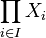# Product-closed property of topological spaces

Jump to: navigation, search
This article defines a metaproperty of topological spaces: a property that can be evaluated to true/false for any property of topological spaces
View a complete list of metaproperties of topological spaces
View topological space properties satisfying this metaproperty| View topological space properties dissatisfying this metaproperty
VIEW RELATED: topological space metaproperty satisfactions| topological space metaproperty dissatisfactions

## Definition

Suppose$\alpha$ is a property of topological spaces, i.e., for any topological space$X$,$X$ either satisfies$\alpha$ or does not satisfy$\alpha$. Then, we say that$\alpha$ is a product-closed property of topological spaces if for any (possibly finite, possibly infinite) collection of topological spaces$X_i, i \in I$, all of which satisfy$\alpha$, the product space$\prod_{i \in I} X_i$, endowed with the product topology, also satisfies$\alpha$.

## Relation with other metaproperties

### Other related metaproperties

To understand the relation between these concepts:

• Examples of properties of topological spaces that are both product-closed and box product-closed: T1 space, Hausdorff space
• Examples of properties that are box product-closed but not product-closed: discrete space
• Examples of properties that are product-closed but not box product-closed: compact space, connected space. Basically this list includes properties that are about smallness or intimacy of some sort. The box topology makes separation easier.